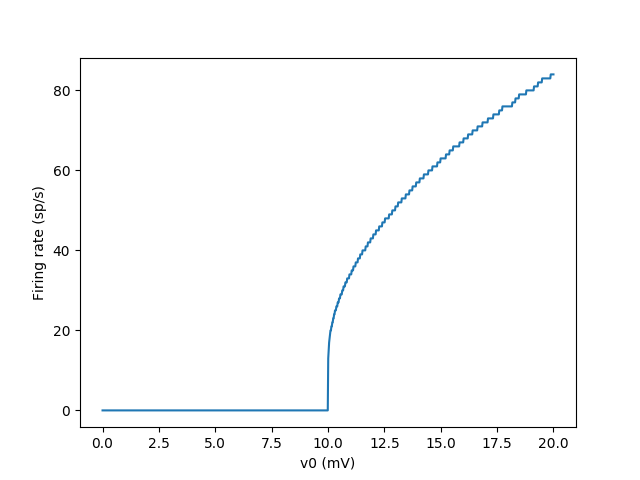# Example: IF_curve_LIF¶

Note

You can launch an interactive, editable version of this example without installing any local files using the Binder service (although note that at some times this may be slow or fail to open):Input-Frequency curve of a IF model.

Network: 1000 unconnected integrate-and-fire neurons (leaky IF) with an input parameter v0. The input is set differently for each neuron.

```from brian2 import *

n = 1000
duration = 1*second
tau = 10*ms
eqs = '''
dv/dt = (v0 - v) / tau : volt (unless refractory)
v0 : volt
'''
group = NeuronGroup(n, eqs, threshold='v > 10*mV', reset='v = 0*mV',
refractory=5*ms, method='exact')
group.v = 0*mV
group.v0 = '20*mV * i / (n-1)'

monitor = SpikeMonitor(group)

run(duration)
plot(group.v0/mV, monitor.count / duration)
xlabel('v0 (mV)')
ylabel('Firing rate (sp/s)')
show()
```